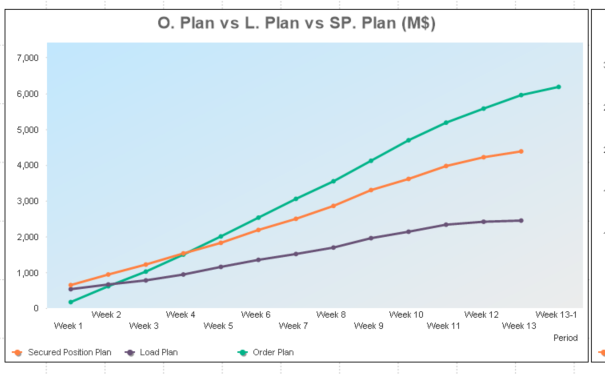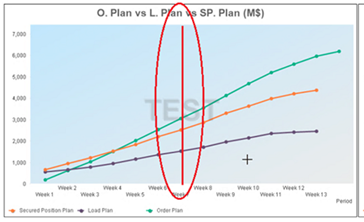# QlikView App Development

Discussion Board for collaboration related to QlikView App Development.

Announcements
Save the Date: QlikWorld Online, June 24-25, 2020. Free global virtual event for data integration and data analytic gurus. Register Today
Highlighted
New Contributor III

## Reference Line in Combo chart

Hi everyone,

I got this situation,

Need to draw a vertical line in my current week (i.e. second image), my x-axis is a string filed name: Period. I'ven trying with reference lines and clicking in Axes, Continuous but i recive the message "no numeric values on x-axis".And i have this formula to know wich is current week:

=if(('W'  & num((date(today())-\$(vQuarterDate)+1)/7,'#0'))='W1','Week 1',

if(('W'  & num((date(today())-\$(vQuarterDate)+1)/7,'#0'))='W2','Week 2',

if(('W'  & num((date(today())-\$(vQuarterDate)+1)/7,'#0'))='W3','Week 3',

if(('W'  & num((date(today())-\$(vQuarterDate)+1)/7,'#0'))='W4','Week 4',

if(('W'  & num((date(today())-\$(vQuarterDate)+1)/7,'#0'))='W5','Week 5',

if(('W'  & num((date(today())-\$(vQuarterDate)+1)/7,'#0'))='W6','Week 6',

if(('W'  & num((date(today())-\$(vQuarterDate)+1)/7,'#0'))='W7','Week 7',

if(('W'  & num((date(today())-\$(vQuarterDate)+1)/7,'#0'))='W8','Week 8',

if(('W'  & num((date(today())-\$(vQuarterDate)+1)/7,'#0'))='W9','Week 9',

if(('W'  & num((date(today())-\$(vQuarterDate)+1)/7,'#0'))='W10','Week 10',

if(('W'  & num((date(today())-\$(vQuarterDate)+1)/7,'#0'))='W11','Week 11',

if(('W'  & num((date(today())-\$(vQuarterDate)+1)/7,'#0'))='W12','Week 12',

if(('W'  & num((date(today())-\$(vQuarterDate)+1)/7,'#0'))='W13','Week 13',

if(('W'  & num((date(today())-\$(vQuarterDate)+1)/7,'#0'))='W14','Week 13-1'))))))))))))))

vQuarterDate:

if(num(Month(Today()))=5 or num(Month(Today()))=6 or num(Month(Today()))=7,Year(Today())&'-05-01',

if(num(Month(Today()))=8 or num(Month(Today()))=9 or num(Month(Today()))=10,Year(Today())&'-08-01',

if(num(Month(Today()))=2 or num(Month(Today()))=3 or num(Month(Today()))=4,Year(Today())&'-02-01',

if(num(Month(Today()))=11 or num(Month(Today()))=12 or num(Month(Today()))=1,Year(Today())&'-11-01'))))

3 Replies
Highlighted
Honored Contributor II

## Re: Reference Line in Combo chart

Do you have continous axis enabled?

New Contributor III

## Re: Reference Line in Combo chart

Yes, and i recive the message "no numeric values on x-axis". due to my string dimension

Highlighted
Honored Contributor II

## Re: Reference Line in Combo chart

can you share the qvw? Maybe scramble the sensitive fields (settings-->document properties --> Scrambling)